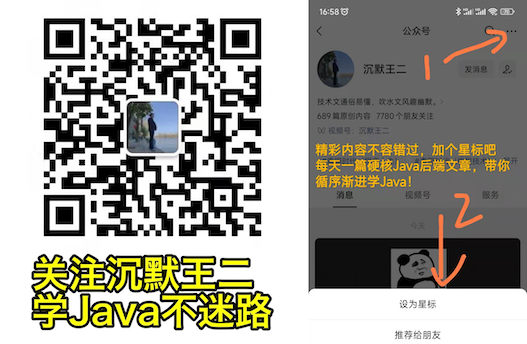# Java 数据类型转换（强制类型转换+自动类型转换）

2022年8月23日

## # 自动类型转换

• 两种数据类型彼此兼容
• 目标类型的取值范围大于源数据类型（低级类型数据转换成高级类型数据）

• 数值型数据的转换：byte→short→int→long→float→double。
• 字符型转换为整型：char→int。

``````public static void main(String[] args) {
float price1 = 10.9f; // 定义牙膏的价格
double price2 = 5.8; // 定义面巾纸的价格
int num1 = 2; // 定义牙膏的数量
int num2 = 4; // 定义面巾纸的数量
double res = price1 * num1 + price2 * num2; // 计算总价
System.out.println("一共付给收银员" + res + "元"); // 输出总价
}
``````

``````一共付给收银员44.99999923706055元
``````

``````byte b = 50;

b = b * 2; // Type mismatch: cannot convert from int to byte
``````

``````byte b = 50;

b = (byte)(b*2);
``````

## # 强制类型转换

``````(type)variableName
``````

``````int a = 3;
double b = 5.0;
a = (int)b;
``````

``````public static void main(String[] args) {
float price1 = 10.9f;
double price2 = 5.8;
int num1 = 2;
int num2 = 4;
int res2 = (int) (price1 * num1 + price2 * num2);
System.out.println("一共付给收银员" + res2 + "元");
}
``````

``````一共付给收银员44元
``````